Short questions on electrostatics for CBSE Board

In this page we have Short questions on electrostatics for CBSE Board . Hope you like them and do not forget to like , social share and comment at the end of the page.
Question 1)  Can we give any amount of charge to a capacitor?
Answer: No, on giving charge continuously to a capacitor, the p.d across its plates will go on rising and ultimately the insulation of the medium between the plates will break. Then the capacitor will be discharged giving sparking
Question 2)  A capacitor is connected to a battery. If we move its plates further apart, work will be done against the electrostatic attraction between the plates. What will be the effect on the energy of the capacitor?
Solution: Since the battery remains connected, the potential difference V between the plates of the capacitor will remain constant.
Now Since Capacitance depends on the distance between the plates, it will decrease with the movement.
Now we know that
Q=CV
Now C is decreasing and V remains constant, the charge will decrease on the capacitor.
Some charges from plates passing through the connecting wire will charge the battery.
Thus some energy of the capacitor will be transferred to the battery. So energy of the capacitor will decrease.
The work done done in moving apart the plates will be used up in this transference and dissipated in the form of heat in the connecting wires
Question 3) two identical metallic spheres A and B of exactly equal masses are taken. Sphere A is given positive charge of Q coulomb and B is given an equal negative charge.So initially before the charge is given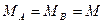What will be the masses after the charging?
a)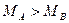b)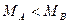c)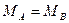d) Nothing can be said
Solution:
The process of giving positive charge involves removal of electrons and that of negative charge involves addition of electrons. Hence the mass of the positively charged sphere will be less than that of negatively charged sphere
Question 4) Two statements
Statement I: A small metallic sphere is placed at the center of a large charged spherical shell and two are the connected by a wire. The charge will not flow from outer sphere to inner sphere
Statement II:  A charged conductor is placed inside the hollow conductor and two are connected by the wire. The whole charge will flow on the outer surface of the outer conductor
1) Both the statement I and II are correct
2) Statement I is correct only
3) Statement II is correct only
4) Both the statement is true
Solution (a)
Question 5) Superposition principle can not be applied to these quantities in electrostatics
a) Electrostatic energy
b) Electric Field
c) Electric Potential
d) Electric force
Solution (a)
It can be applied to electric Field, electric potential and electric force
Question 6)  What is electrostatic Shielding?
Solution The absence of electric field inside the charged conductors means that electric lines of force cannot enter the empty space of any hollow conductor. Thus hollow conductor can be used to acts as electrostatic shield. So sensitive electric instruments can be saved from external electric fields by covering them with hollow conductor.
Question 7)
Consider the electric field at the points A, B, C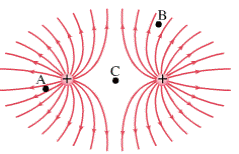List the points in increasing order of electric field strength
a) C> B>A
b) C>A>B
c) A>B>C
d) None of the
Solution (a)

Question 8) Consider the system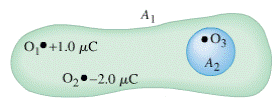Which of the following is true?
a) Electric flux through surface A2 is zero
b) Electric Flux through surface A1 is not zero
c) Electric field at the surface A2 is not zero
d) None of these
Solution (a),(b) and (c)
Question 9)  Two conducting charged spheres X and Y having unequal charges are connected by the wire. Which of the following is true?
a) Charge is conserved
b) Electrostatic energy is conserved
c) Both the charge and electrostatic energy is conserved
d) Neither of these is conserved
Solution (a)
Question 10)  What is true of equipotential surface?
a) The PD between any two points on the surface is zero
b) The electric field is always perpendicular to the surface
c) Equipotential surface are always spherical
d) No work is done in moving a charge along the surface
Solution (a) (b) and (d)
 Electric Generators Electric Motors Device which converts mechanical energy into electrical energy Device which converts electrical energy into mechanical energy It is based on Electromagnetic Induction Principle It  is based on moving coil galvanometer When a closed coil is rotated rapidly in a strong magnetic field ,the magnetic flux through the coil changes continuously .Hence an EMF is induced in the coil and current flows in it in a direction by Fleming right hand rule When a current is passed through a coil in a magnetic field ,the coil experiences a torque in the direction given by Fleming left hand rule. This torque  gives a continuous rotatory motion in the coil in the magnetic field Two types AC dynamo and DC dynamo Back Emf is also generated in Motor since it cuts the magnetic flux of the field magnet